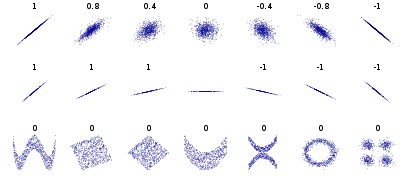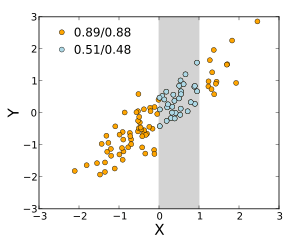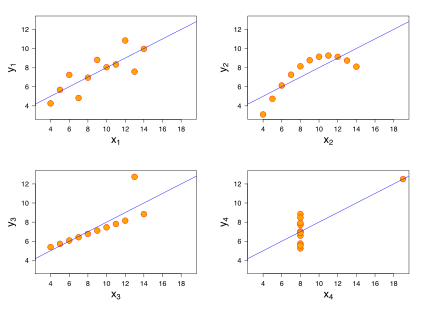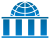# Correlation and dependence

In statistics, dependence or association is any statistical relationship, whether causal or not, between two random variables or two sets of data. Correlation is any of a broad class of statistical relationships involving dependence, though in common usage it most often refers to the extent to which two variables have a linear relationship with each other. Familiar examples of dependent phenomena include the correlation between the physical statures of parents and their offspring, and the correlation between the demand for a product and its price.

Correlations are useful because they can indicate a predictive relationship that can be exploited in practice. For example, an electrical utility may produce less power on a mild day based on the correlation between electricity demand and weather. In this example there is a causal relationship, because extreme weather causes people to use more electricity for heating or cooling; however, correlation is not sufficient to demonstrate the presence of such a causal relationship (i.e., correlation does not imply causation).

Formally, dependence refers to any situation in which random variables do not satisfy a mathematical condition of probabilistic independence. In loose usage, correlation can refer to any departure of two or more random variables from independence, but technically it refers to any of several more specialized types of relationship between mean values. There are several correlation coefficients, often denoted ρ or r, measuring the degree of correlation. The most common of these is the Pearson correlation coefficient, which is sensitive only to a linear relationship between two variables (which may exist even if one is a nonlinear function of the other). Other correlation coefficients have been developed to be more robust than the Pearson correlation – that is, more sensitive to nonlinear relationships. Mutual information can also be applied to measure dependence between two variables.Several sets of (x, y) points, with the Pearson correlation coefficient of x and y for each set. Note that the correlation reflects the noisiness and direction of a linear relationship (top row), but not the slope of that relationship (middle), nor many aspects of nonlinear relationships (bottom). N.B.: the figure in the center has a slope of 0 but in that case the correlation coefficient is undefined because the variance of Y is zero.

## Pearson's product-moment coefficient

The most familiar measure of dependence between two quantities is the Pearson product-moment correlation coefficient, or "Pearson's correlation coefficient", commonly called simply "the correlation coefficient". It is obtained by dividing the covariance of the two variables by the product of their standard deviations. Karl Pearson developed the coefficient from a similar but slightly different idea by Francis Galton.

The population correlation coefficient ρX,Y between two random variables X and Y with expected values μX and μY and standard deviations σX and σY is defined as:where E is the expected value operator, cov means covariance, and corr is a widely used alternative notation for the correlation coefficient.

The Pearson correlation is defined only if both of the standard deviations are finite and nonzero. It is a corollary of the Cauchy–Schwarz inequality that the correlation cannot exceed 1 in absolute value. The correlation coefficient is symmetric: corr(X,Y) = corr(Y,X).

The Pearson correlation is +1 in the case of a perfect direct (increasing) linear relationship (correlation), −1 in the case of a perfect decreasing (inverse) linear relationship (anticorrelation), and some value in the open interval (−1, 1) in all other cases, indicating the degree of linear dependence between the variables. As it approaches zero there is less of a relationship (closer to uncorrelated). The closer the coefficient is to either −1 or 1, the stronger the correlation between the variables.

If the variables are independent, Pearson's correlation coefficient is 0, but the converse is not true because the correlation coefficient detects only linear dependencies between two variables. For example, suppose the random variable X is symmetrically distributed about zero, and Y = X2. Then Y is completely determined by X, so that X and Y are perfectly dependent, but their correlation is zero; they are uncorrelated. However, in the special case when X and Y are jointly normal, uncorrelatedness is equivalent to independence.

If we have a series of n measurements of X and Y written as xi and yi for i = 1, 2, ..., n, then the sample correlation coefficient can be used to estimate the population Pearson correlation r between X and Y. The sample correlation coefficient is written:where x and y are the sample means of X and Y, and sx and sy are the sample standard deviations of X and Y.

This can also be written as:If x and y are results of measurements that contain measurement error, the realistic limits on the correlation coefficient are not −1 to +1 but a smaller range.

For the case of a linear model with a single independent variable, the coefficient of determination (R squared) is the square of r, Pearson's product-moment coefficient.

## Rank correlation coefficients

Rank correlation coefficients, such as Spearman's rank correlation coefficient and Kendall's rank correlation coefficient (τ) measure the extent to which, as one variable increases, the other variable tends to increase, without requiring that increase to be represented by a linear relationship. If, as the one variable increases, the other decreases, the rank correlation coefficients will be negative. It is common to regard these rank correlation coefficients as alternatives to Pearson's coefficient, used either to reduce the amount of calculation or to make the coefficient less sensitive to non-normality in distributions. However, this view has little mathematical basis, as rank correlation coefficients measure a different type of relationship than the Pearson product-moment correlation coefficient, and are best seen as measures of a different type of association, rather than as alternative measure of the population correlation coefficient.

To illustrate the nature of rank correlation, and its difference from linear correlation, consider the following four pairs of numbers (x, y):

(0, 1), (10, 100), (101, 500), (102, 2000).

As we go from each pair to the next pair x increases, and so does y. This relationship is perfect, in the sense that an increase in x is always accompanied by an increase in y. This means that we have a perfect rank correlation, and both Spearman's and Kendall's correlation coefficients are 1, whereas in this example Pearson product-moment correlation coefficient is 0.7544, indicating that the points are far from lying on a straight line. In the same way if y always decreases when x increases, the rank correlation coefficients will be −1, while the Pearson product-moment correlation coefficient may or may not be close to −1, depending on how close the points are to a straight line. Although in the extreme cases of perfect rank correlation the two coefficients are both equal (being both +1 or both −1), this is not generally the case, and so values of the two coefficients cannot meaningfully be compared. For example, for the three pairs (1, 1) (2, 3) (3, 2) Spearman's coefficient is 1/2, while Kendall's coefficient is 1/3.

## Other measures of dependence among random variables

The information given by a correlation coefficient is not enough to define the dependence structure between random variables. The correlation coefficient completely defines the dependence structure only in very particular cases, for example when the distribution is a multivariate normal distribution. (See diagram above.) In the case of elliptical distributions it characterizes the (hyper-)ellipses of equal density, however, it does not completely characterize the dependence structure (for example, a multivariate t-distribution's degrees of freedom determine the level of tail dependence).

Distance correlation was introduced to address the deficiency of Pearson's correlation that it can be zero for dependent random variables; zero distance correlation implies independence.

The Randomized Dependence Coefficient is a computationally efficient, copula-based measure of dependence between multivariate random variables. RDC is invariant with respect to non-linear scalings of random variables, is capable of discovering a wide range of functional association patterns and takes value zero at independence.

The correlation ratio is able to detect almost any functional dependency, and the entropy-based mutual information, total correlation and dual total correlation are capable of detecting even more general dependencies. These are sometimes referred to as multi-moment correlation measures, in comparison to those that consider only second moment (pairwise or quadratic) dependence.

The polychoric correlation is another correlation applied to ordinal data that aims to estimate the correlation between theorised latent variables.

One way to capture a more complete view of dependence structure is to consider a copula between them.

The coefficient of determination generalizes the correlation coefficient for relationships beyond simple linear regression.

## Sensitivity to the data distribution

The degree of dependence between variables X and Y does not depend on the scale on which the variables are expressed. That is, if we are analyzing the relationship between X and Y, most correlation measures are unaffected by transforming X to a + bX and Y to c + dY, where a, b, c, and d are constants (b and d being positive). This is true of some correlation statistics as well as their population analogues. Some correlation statistics, such as the rank correlation coefficient, are also invariant to monotone transformations of the marginal distributions of X and/or Y.Pearson/Spearman correlation coefficients between X and Y are shown when the two variables' ranges are unrestricted, and when the range of X is restricted to the interval (0,1).

Most correlation measures are sensitive to the manner in which X and Y are sampled. Dependencies tend to be stronger if viewed over a wider range of values. Thus, if we consider the correlation coefficient between the heights of fathers and their sons over all adult males, and compare it to the same correlation coefficient calculated when the fathers are selected to be between 165 cm and 170 cm in height, the correlation will be weaker in the latter case. Several techniques have been developed that attempt to correct for range restriction in one or both variables, and are commonly used in meta-analysis; the most common are Thorndike's case II and case III equations.

Various correlation measures in use may be undefined for certain joint distributions of X and Y. For example, the Pearson correlation coefficient is defined in terms of moments, and hence will be undefined if the moments are undefined. Measures of dependence based on quantiles are always defined. Sample-based statistics intended to estimate population measures of dependence may or may not have desirable statistical properties such as being unbiased, or asymptotically consistent, based on the spatial structure of the population from which the data were sampled.

Sensitivity to the data distribution can be used to an advantage. For example, scaled correlation is designed to use the sensitivity to the range in order to pick out correlations between fast components of time series. By reducing the range of values in a controlled manner, the correlations on long time scale are filtered out and only the correlations on short time scales are revealed.

## Correlation matrices

"Correlation matrix" redirects here. For correlation matrices in quantum physics, see Quark–lepton complementarity.

The correlation matrix of n random variables X1, ..., Xn is the n  ×  n matrix whose i,j entry is corr(Xi, Xj). If the measures of correlation used are product-moment coefficients, the correlation matrix is the same as the covariance matrix of the standardized random variables Xi / σ (Xi) for i = 1, ..., n. This applies to both the matrix of population correlations (in which case "σ" is the population standard deviation), and to the matrix of sample correlations (in which case "σ" denotes the sample standard deviation). Consequently, each is necessarily a positive-semidefinite matrix.

The correlation matrix is symmetric because the correlation between Xi and Xj is the same as the correlation between Xj and Xi.

## Common misconceptions

### Correlation and causality

The conventional dictum that "correlation does not imply causation" means that correlation cannot be used to infer a causal relationship between the variables. This dictum should not be taken to mean that correlations cannot indicate the potential existence of causal relations. However, the causes underlying the correlation, if any, may be indirect and unknown, and high correlations also overlap with identity relations (tautologies), where no causal process exists. Consequently, establishing a correlation between two variables is not a sufficient condition to establish a causal relationship (in either direction).

A correlation between age and height in children is fairly causally transparent, but a correlation between mood and health in people is less so. Does improved mood lead to improved health, or does good health lead to good mood, or both? Or does some other factor underlie both? In other words, a correlation can be taken as evidence for a possible causal relationship, but cannot indicate what the causal relationship, if any, might be.

### Correlation and linearityFour sets of data with the same correlation of 0.816

The Pearson correlation coefficient indicates the strength of a linear relationship between two variables, but its value generally does not completely characterize their relationship. In particular, if the conditional mean of Y given X, denoted E(Y | X), is not linear in X, the correlation coefficient will not fully determine the form of E(Y | X).

The image on the right shows scatter plots of Anscombe's quartet, a set of four different pairs of variables created by Francis Anscombe. The four y variables have the same mean (7.5), variance (4.12), correlation (0.816) and regression line (y = 3 + 0.5x). However, as can be seen on the plots, the distribution of the variables is very different. The first one (top left) seems to be distributed normally, and corresponds to what one would expect when considering two variables correlated and following the assumption of normality. The second one (top right) is not distributed normally; while an obvious relationship between the two variables can be observed, it is not linear. In this case the Pearson correlation coefficient does not indicate that there is an exact functional relationship: only the extent to which that relationship can be approximated by a linear relationship. In the third case (bottom left), the linear relationship is perfect, except for one outlier which exerts enough influence to lower the correlation coefficient from 1 to 0.816. Finally, the fourth example (bottom right) shows another example when one outlier is enough to produce a high correlation coefficient, even though the relationship between the two variables is not linear.

These examples indicate that the correlation coefficient, as a summary statistic, cannot replace visual examination of the data. Note that the examples are sometimes said to demonstrate that the Pearson correlation assumes that the data follow a normal distribution, but this is not correct.

## Bivariate normal distribution

If a pair (X, Y) of random variables follows a bivariate normal distribution, the conditional mean E(X|Y) is a linear function of Y, and the conditional mean E(Y|X) is a linear function of X. The correlation coefficient r between X and Y, along with the marginal means and variances of X and Y, determines this linear relationship:whereandare the expected values of X and Y, respectively, and σx and σy are the standard deviations of X and Y, respectively.

## Partial correlation

Main article: Partial correlation

If a population or data-set is characterized by more than two variables, a partial correlation coefficient measures the strength of dependence between a pair of variables that is not accounted for by the way in which they both change in response to variations in a selected subset of the other variables.

Further information: Correlation (disambiguation)
1. Croxton, Frederick Emory; Cowden, Dudley Johnstone; Klein, Sidney (1968) Applied General Statistics, Pitman. ISBN 9780273403159 (page 625)
2. Dietrich, Cornelius Frank (1991) Uncertainty, Calibration and Probability: The Statistics of Scientific and Industrial Measurement 2nd Edition, A. Higler. ISBN 9780750300605 (Page 331)
3. Aitken, Alexander Craig (1957) Statistical Mathematics 8th Edition. Oliver & Boyd. ISBN 9780050013007 (Page 95)
4. Rodgers, J. L.; Nicewander, W. A. (1988). "Thirteen ways to look at the correlation coefficient". The American Statistician. 42 (1): 59–66. doi:10.1080/00031305.1988.10475524. JSTOR 2685263.
5. Dowdy, S. and Wearden, S. (1983). "Statistics for Research", Wiley. ISBN 0-471-08602-9 pp 230
6. Francis, DP; Coats AJ; Gibson D (1999). "How high can a correlation coefficient be?". Int J Cardiol. 69 (2): 185–199. doi:10.1016/S0167-5273(99)00028-5.
7. Yule, G.U and Kendall, M.G. (1950), "An Introduction to the Theory of Statistics", 14th Edition (5th Impression 1968). Charles Griffin & Co. pp 258–270
8. Kendall, M. G. (1955) "Rank Correlation Methods", Charles Griffin & Co.
9. Mahdavi Damghani B. (2013). "The Non-Misleading Value of Inferred Correlation: An Introduction to the Cointelation Model". Wilmott Magazine. doi:10.1002/wilm.10252.
10. Székely, G. J. Rizzo; Bakirov, N. K. (2007). "Measuring and testing independence by correlation of distances". Annals of Statistics. 35 (6): 2769–2794. doi:10.1214/009053607000000505.
11. Székely, G. J.; Rizzo, M. L. (2009). "Brownian distance covariance". Annals of Applied Statistics. 3 (4): 1233–1303. doi:10.1214/09-AOAS312.
12. Lopez-Paz D. and Hennig P. and Schölkopf B. (2013). "The Randomized Dependence Coefficient", "Conference on Neural Information Processing Systems" Reprint
13. Thorndike, Robert Ladd (1947). Research problems and techniques (Report No. 3). Washington DC: US Govt. print. off.
14. Nikolić, D; Muresan, RC; Feng, W; Singer, W (2012). "Scaled correlation analysis: a better way to compute a cross-correlogram". European Journal of Neuroscience: 1–21. doi:10.1111/j.1460-9568.2011.07987.x.
15. Aldrich, John (1995). "Correlations Genuine and Spurious in Pearson and Yule". Statistical Science. 10 (4): 364–376. doi:10.1214/ss/1177009870. JSTOR 2246135.
16. Mahdavi Damghani, Babak (2012). "The Misleading Value of Measured Correlation". Wilmott. 2012 (1): 64–73. doi:10.1002/wilm.10167.
17. Anscombe, Francis J. (1973). "Graphs in statistical analysis". The American Statistician. 27: 17–21. doi:10.2307/2682899. JSTOR 2682899.
• Cohen, J., Cohen P., West, S.G., & Aiken, L.S. (2002). Applied multiple regression/correlation analysis for the behavioral sciences (3rd ed.). Psychology Press. ISBN 0-8058-2223-2.
• Hazewinkel, Michiel, ed. (2001), "Correlation (in statistics)", Encyclopedia of Mathematics, Springer, ISBN 978-1-55608-010-4
• Oestreicher, J. & D. R. (February 26, 2015). Plague of Equals: A science thriller of international disease, politics and drug discovery. California: Omega Cat Press. p. 408. ISBN 978-0963175540.Look up correlation in Wiktionary, the free dictionary.Look up dependence in Wiktionary, the free dictionary.Wikimedia Commons has media related to Correlation and dependence.Wikiversity has learning materials about Correlation